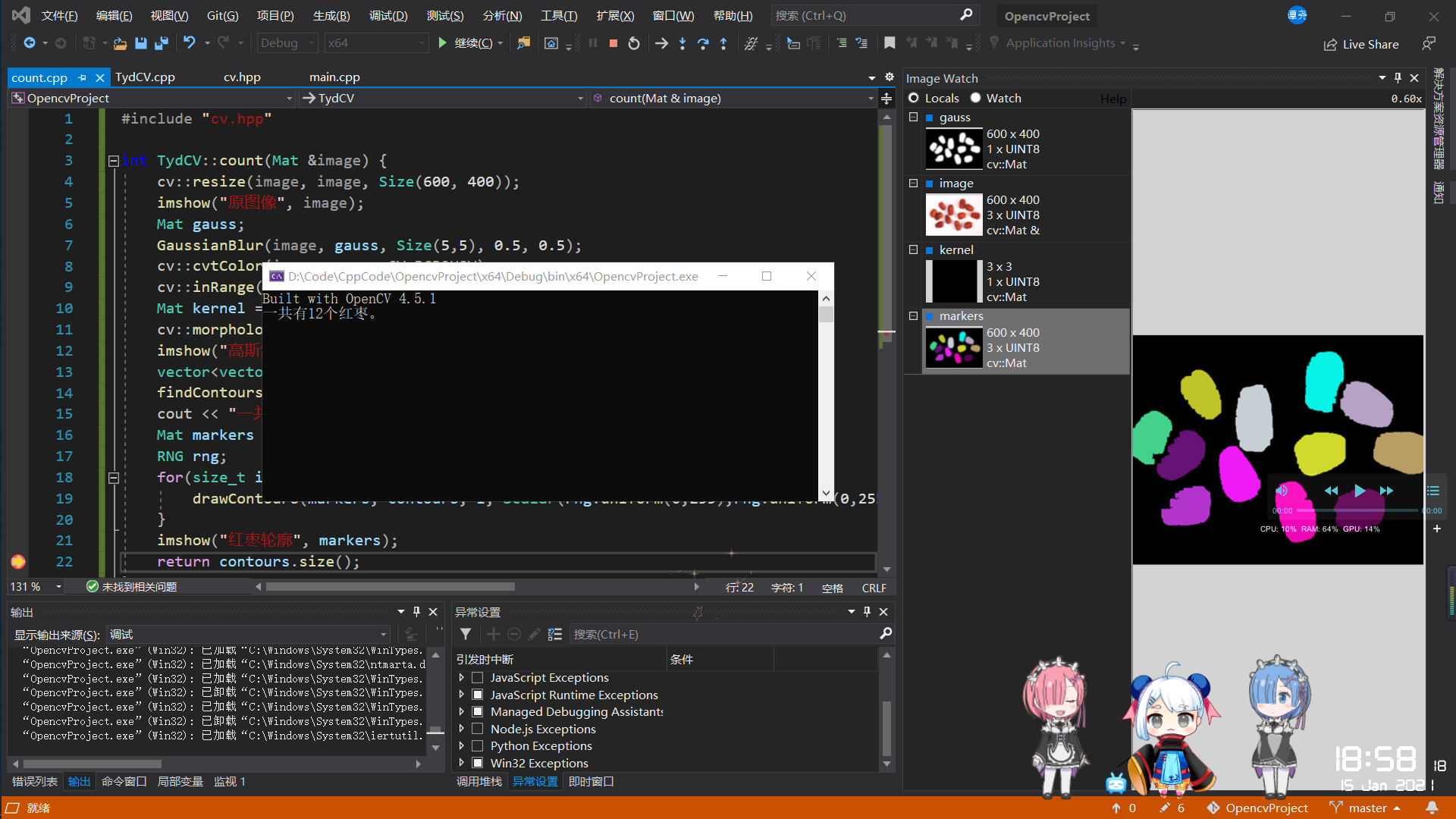### C++ OpenCV利用颜色分割来数红枣

#### 一杯茶一包烟12个红枣数一天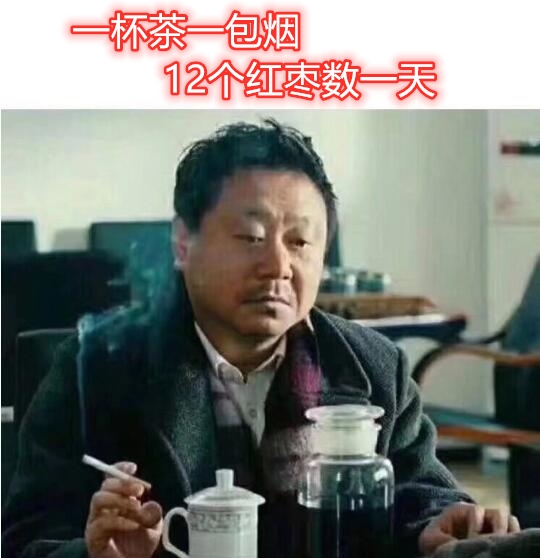#### 加载红枣图像

``````string IMG_PATH = "D:/Code/CppCode/OpencvProject/img/";
cv::resize(image, image, Size(600, 400));
imshow("原图像", image);
``````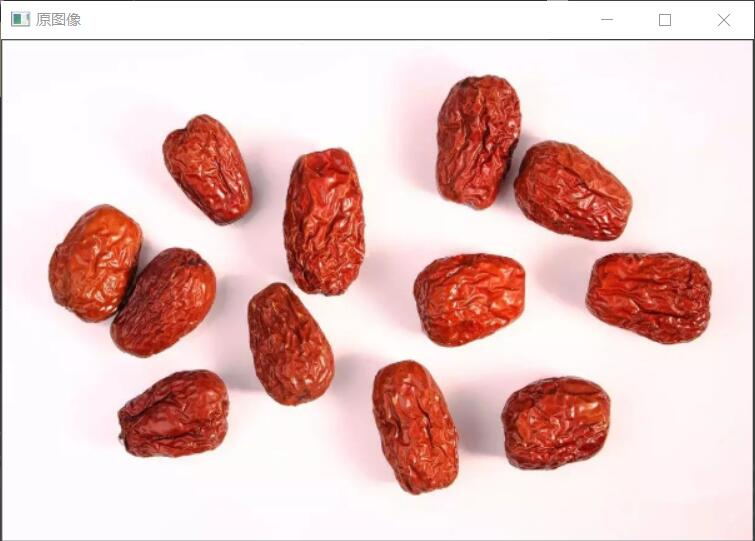#### 高斯滤波，定义颜色并利用HSV进行颜色分割

``````void GaussianBlur( InputArray src, OutputArray dst, Size ksize, double sigmaX, double sigmaY = 0, int borderType = BORDER_DEFAULT )
``````

``````void cvtColor( InputArray src, OutputArray dst, int code, int dstCn = 0 )
``````

``````    void inRange(InputArray src, InputArray lowerb, InputArray upperb, OutputArray dst)
``````

``````Mat gauss;
GaussianBlur(image, gauss, Size(5,5), 0.5, 0.5);
cv::cvtColor(image, gauss,CV_BGR2HSV);
cv::inRange(gauss, Scalar(0,43,46), Scalar(10,255,255), gauss);
``````

#### 形态学操作，我做了四次开操作

morphologyEx这个函数可以用来对图像进行一系列的膨胀腐蚀组合，在C++中 morphologyEx 函数定义如下：

``````void morphologyEx( InputArray src, OutputArray dst, int op, InputArray kernel, Point anchor = Point(-1,-1), int iterations = 1, int borderType = BORDER_CONSTANT, const Scalar& borderValue = morphologyDefaultBorderValue() )
``````

``````enum MorphTypes{
MORPH_ERODE = 0, //腐蚀，跟erode函数的腐蚀效果一样
MORPH_DILATE = 1, //膨胀，跟dilate函数的膨胀效果一样
MORPH_OPEN = 2, //开操作，实质是先腐蚀后膨胀的操作
MORPH_CLOSE = 3, //闭操作，实质上是先膨胀后腐蚀的操作
MORPH_TOPHAT = 5, //顶帽操作
MORPH_BLACKHAT = 6, //黑帽操作
MORPH_HITMISS = 7  //命中或未命中
};
``````

``````Mat getStructuringElement(int shape, Size esize, Point anchor = Point(-1, -1))
``````

``````Mat kernel = getStructuringElement(MORPH_RECT, Size(3,3));
cv::morphologyEx(gauss, gauss, MORPH_OPEN, kernel, Point(-1,-1), 4);
imshow("高斯滤波形态学处理后的图像", gauss);
``````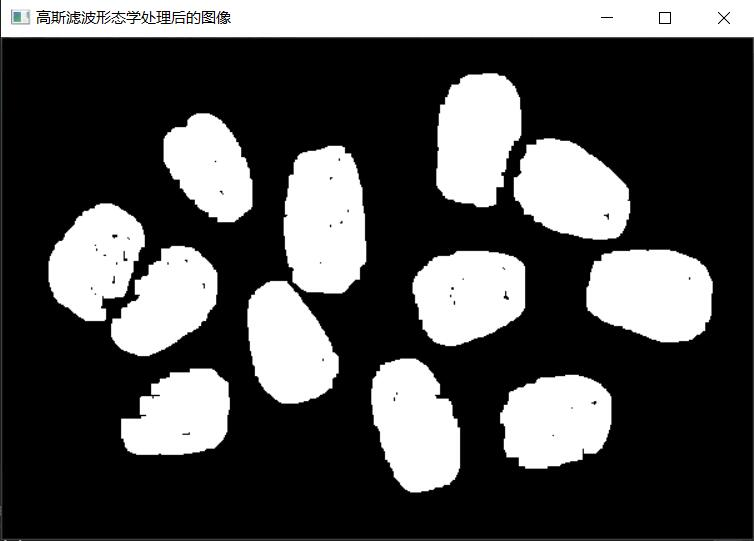#### 寻找轮廓并输出轮廓个数

OpenCV中，轮廓是由STL风格的vector<>模板对象表示的，其中vector中的每个元素都编码了曲线上，下一点的位置信息。 查找图像轮廓的函数是findContours()，在C++中 findContours() 有两种函数定义：

``````void findContours(
cv::InputOutputArray image, // 输入的8位单通道“二值”图像
cv::OutputArrayOfArrays contours, // 包含points的vectors的vector
cv::OutputArray hierarchy, // (可选) 拓扑信息
int mode, // 轮廓检索模式
int method, // 轮廓近似方法
cv::Point offset = cv::Point() // (可选) 所有点的偏移
);
void findContours(
cv::InputOutputArray image, // 输入的8位单通道“二值”图像
cv::OutputArrayOfArrays contours, // 包含points的vectors的vector
int mode, // 轮廓检索模式
int method, // 轮廓近似方法
cv::Point offset = cv::Point() //  (可选) 所有点的偏移
);
``````

``````enum RetrievalModes {
RETR_EXTERNAL = 0,  //只提取最外面的轮廓
RETR_LIST = 1,    //提取所有轮廓并将其放入列表
RETR_CCOMP = 2,  //提取所有轮廓并将组织成一个两层结构，其中顶层轮廓是外部轮廓，第二层轮廓是“洞”的轮廓
RETR_TREE = 3,  //提取所有轮廓并重建嵌套轮廓的完整层级结构
RETR_FLOODFILL = 4  //填色
}
``````

``````enum ContourApproximationModes {
CHAIN_APPROX_NONE = 1,  //绝对存储所有轮廓点，将轮廓中的所有点的编码转换成点
CHAIN_APPROX_SIMPLE = 2,  //压缩水平，垂直和对角线段，仅保留其端点
CHAIN_APPROX_TC89_L1 = 3,  //应用Teh-Chin链近似算法中的一种风格
CHAIN_APPROX_TC89_KCOS = 4  //应用Teh-Chin链逼近算法的一种风格
}
``````

``````vector<vector<Point>> contours;
findContours(gauss, contours, CV_RETR_EXTERNAL, CV_CHAIN_APPROX_SIMPLE);
cout << "一共有" << contours.size() << "个红枣。" << endl;
``````

#### 画出轮廓并显示图像

drawContours函数主要用于将查找到的轮廓绘制到图像上，即画出图像的轮廓，在C++中 drawContours 函数定义如下：

``````void drawContours(InputOutputArray image, InputArrayOfArrays contours, int contourIdx, const Scalar& color, int thickness=1, int lineType=8, InputArray hierarchy=noArray(), int maxLevel=INT_MAX, Point offset=Point() )
``````

``````Mat markers = Mat::zeros(image.size(), CV_8UC3);
RNG rng;   //Royal Never Give up???
for(size_t i = 0; i < contours.size(); i++) {
drawContours(markers, contours, i, Scalar(rng.uniform(0,255),rng.uniform(0,255),rng.uniform(0,255)), -1, 8);
}
imshow("红枣轮廓", markers);
``````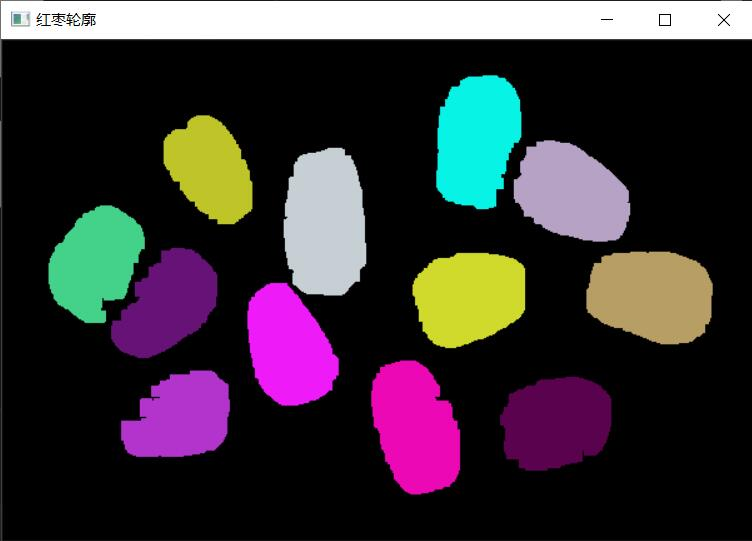#### 最后把数红枣的完整代码写在类函数里面。

``````int TydCV::count(Mat &image) {
cv::resize(image, image, Size(600, 400));
imshow("原图像", image);
Mat gauss;
GaussianBlur(image, gauss, Size(5,5), 0.5, 0.5);
cv::cvtColor(image, gauss,CV_BGR2HSV);
cv::inRange(gauss, Scalar(0,43,46), Scalar(10,255,255), gauss);
Mat kernel = getStructuringElement(MORPH_RECT, Size(3,3));
cv::morphologyEx(gauss, gauss, MORPH_OPEN, kernel, Point(-1,-1), 4);
imshow("高斯滤波形态学处理后的图像", gauss);
vector<vector<Point>> contours;
findContours(gauss, contours, CV_RETR_EXTERNAL, CV_CHAIN_APPROX_SIMPLE);
cout << "一共有" << contours.size() << "个红枣。" << endl;
Mat markers = Mat::zeros(image.size(), CV_8UC3);
RNG rng;
for(size_t i = 0; i < contours.size(); i++) {
drawContours(markers, contours, i, Scalar(rng.uniform(0,255),rng.uniform(0,255),rng.uniform(0,255)), -1, 8);
}
imshow("红枣轮廓", markers);
return contours.size();
}
``````